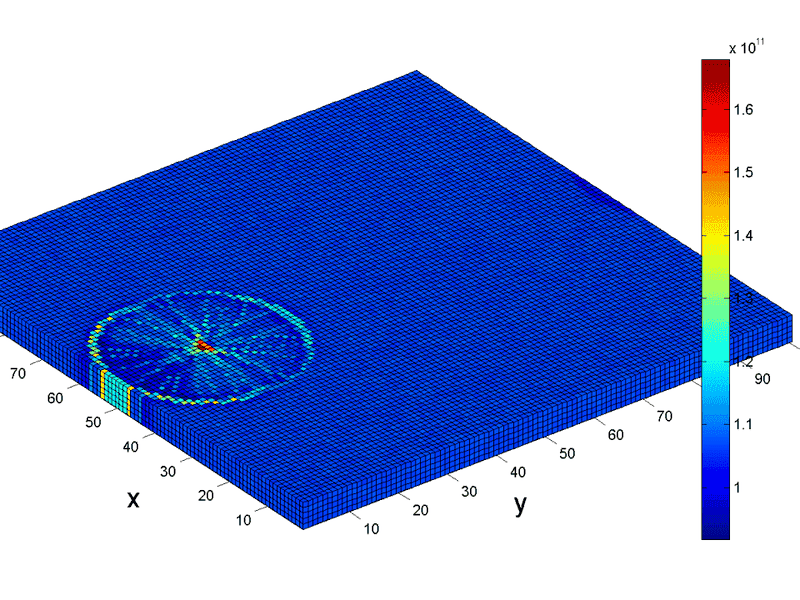# How to set zero value boxes transparent in 3D plots?

• MATLAB
Gold Member
I used a function called ind2patch to make a big 3D block which contains a number of smaller blocks in 3 dimensions. Each small block has a value which the magnitude is represented by a color.One example is shown below:However, most of the boxes have very low or zero value and I don't need to show them. How can I make these boxes transparent? I just want to show the boxes with non-zero value.

For the above 3D plot I use a matrix M to store the value of each box (i,j,k) and use the following algorithm to plot:

indPatch=1:numel(M);
[F,V,C]=ind2patch(indPatch,M,'v'); %Call the function ind2patch in order to plot 3D cube with color

xlabel('y','fontsize',20);ylabel('x','fontsize',20); zlabel('z','fontsize',20); hold on;
set(get(gca,'xlabel'),'Position',[5 -50 30]); %set position of axis label
set(get(gca,'ylabel'),'Position',[5 50 -15]);
set(get(gca,'zlabel'),'Position',[64 190 -60]);
patch('Faces',F,'Vertices',V,'FaceColor','flat','CData',C,'EdgeColor','k','FaceAlpha',0.5);
axis equal; view(3); axis tight; axis vis3d; grid off;